Learn about Sets on our Youtube Channel - https://you.tube/Chapter-1-Class-11-Sets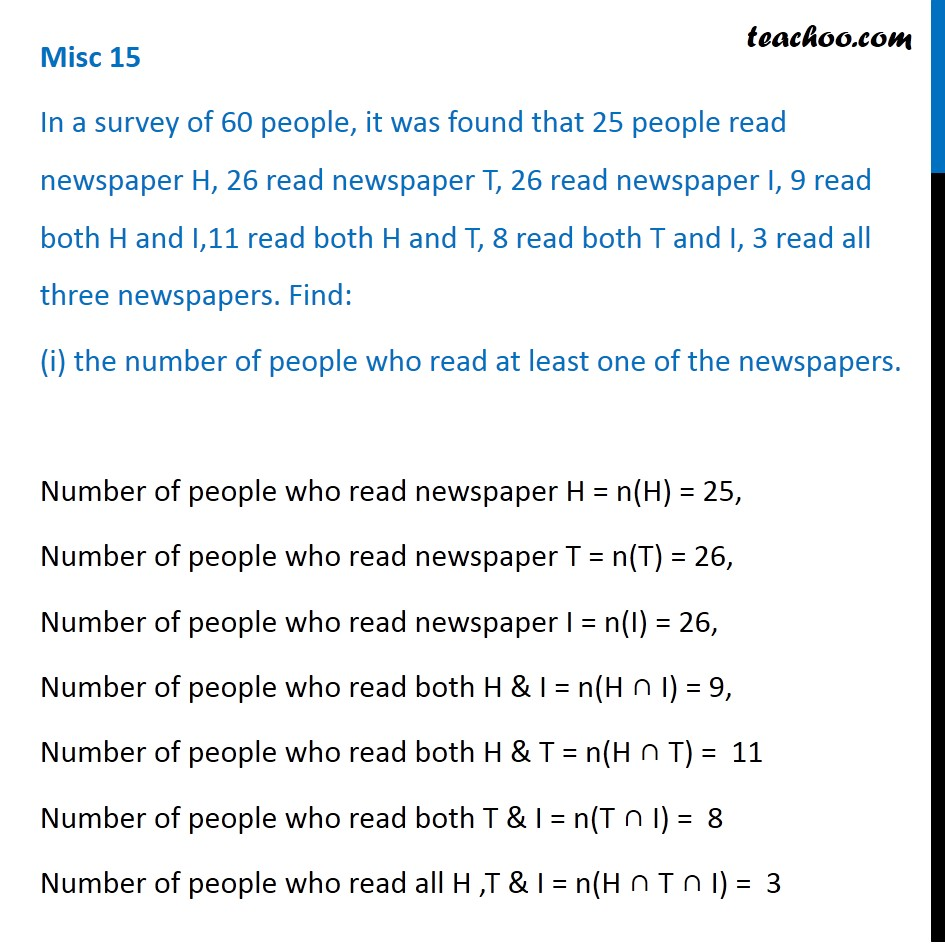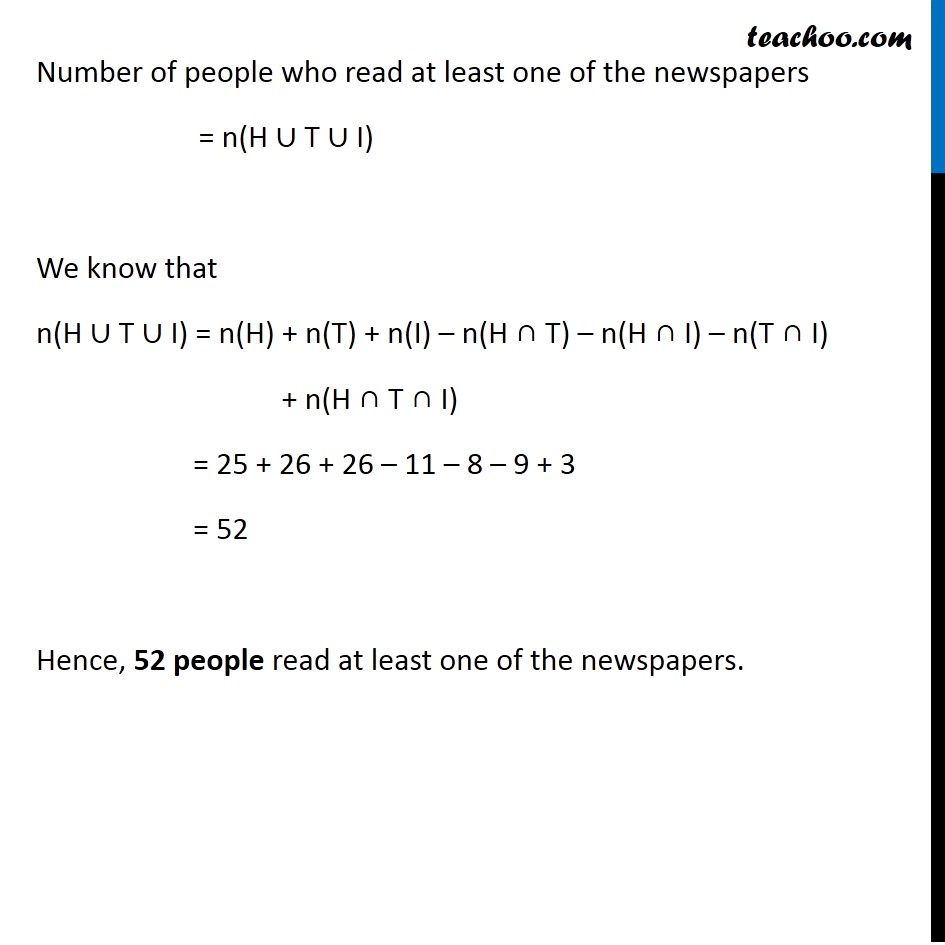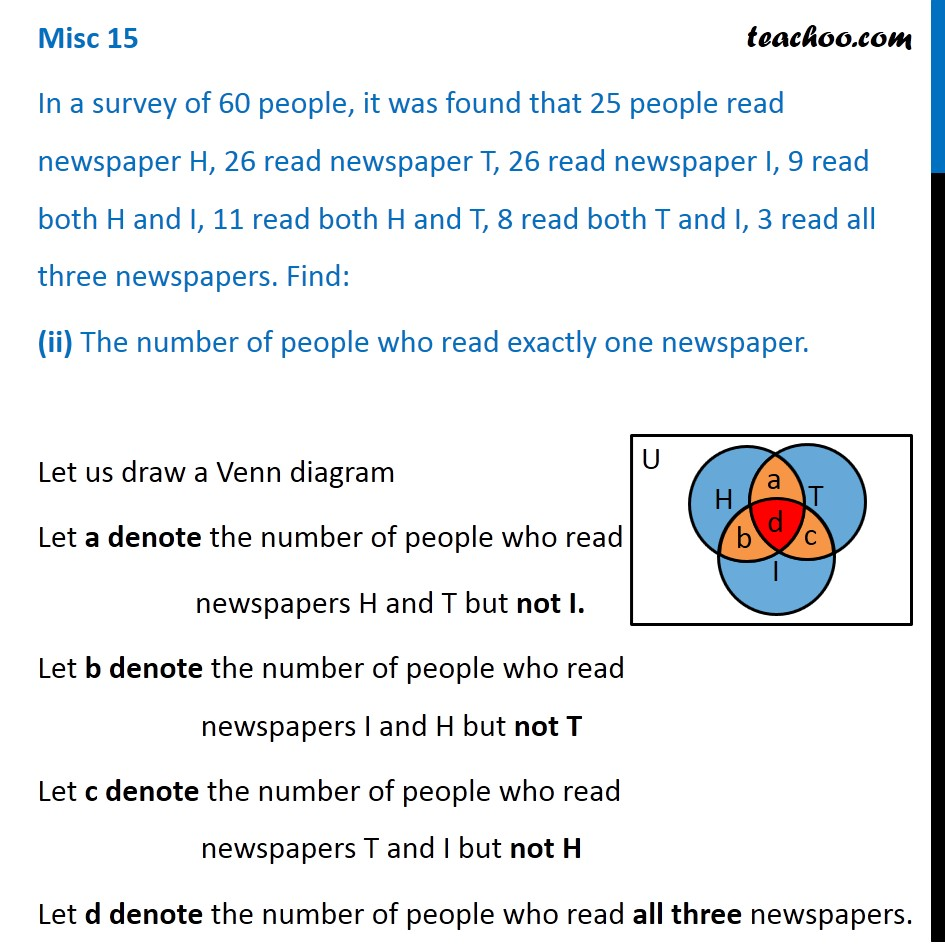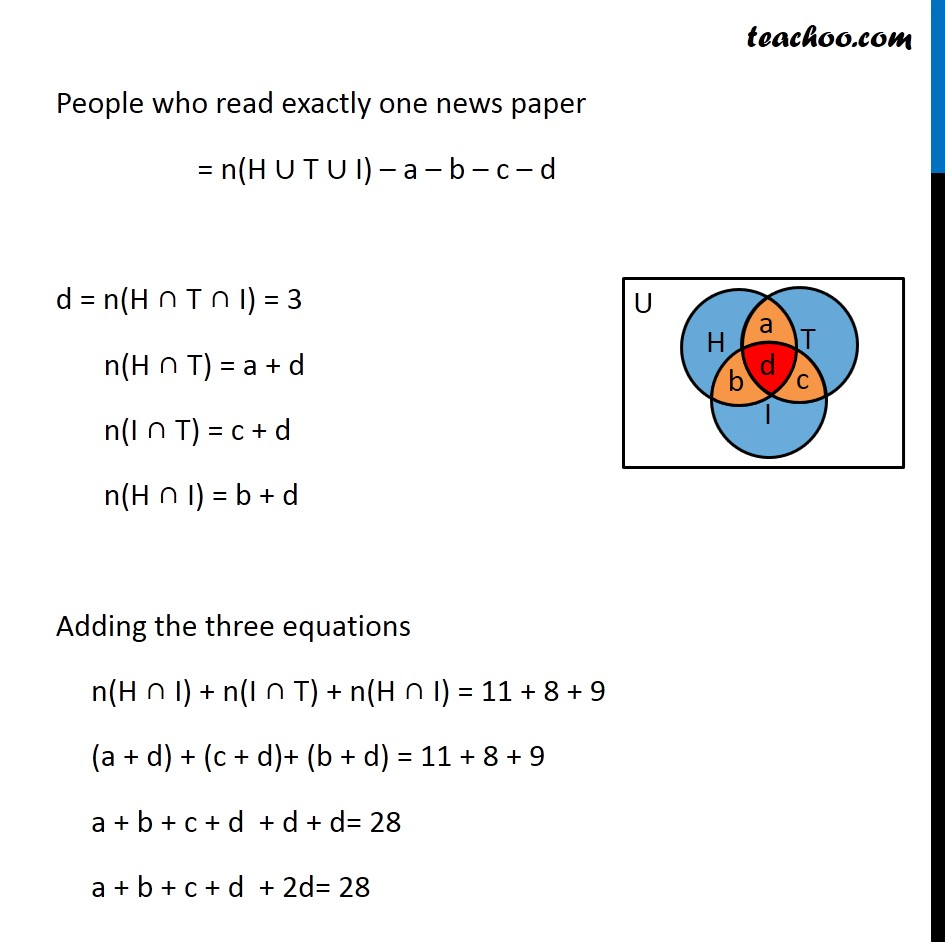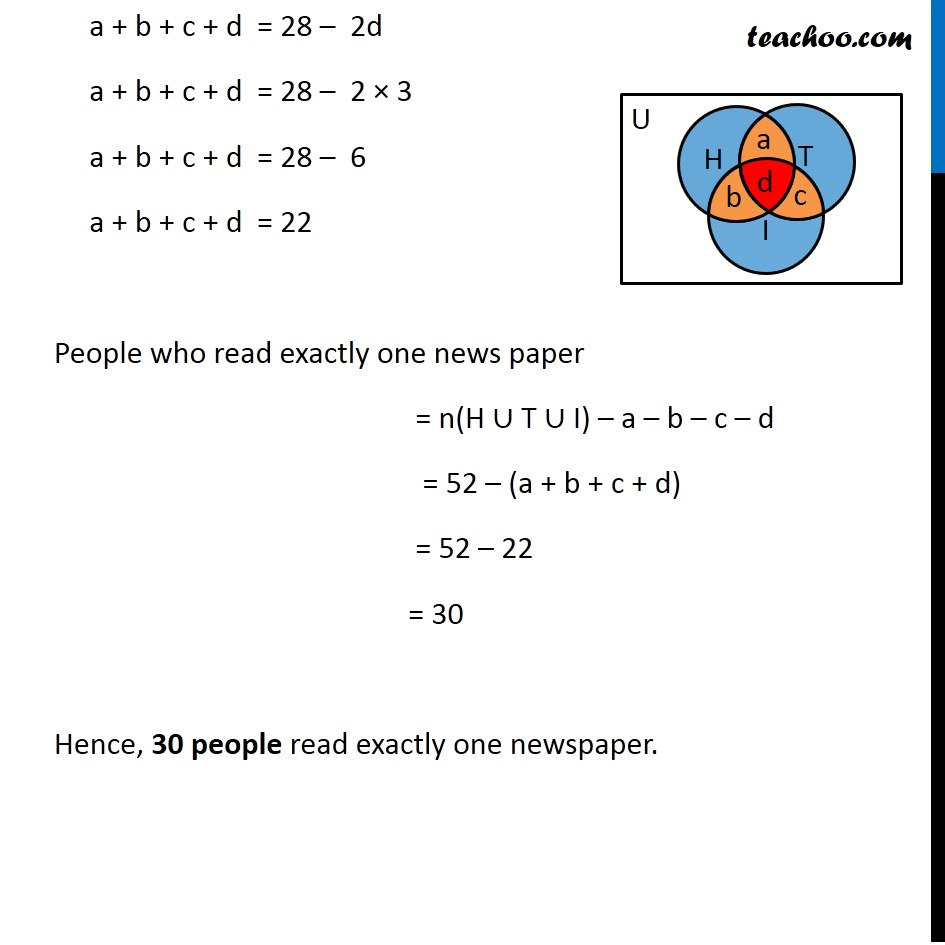1. Chapter 1 Class 11 Sets
2. Serial order wise
3. Miscellaneous

Transcript

Misc 15 In a survey of 60 people, it was found that 25 people read newspaper H, 26 read newspaper T, 26 read newspaper I, 9 read both H and I,11 read both H and T, 8 read both T and I, 3 read all three newspapers. Find: (i) the number of people who read at least one of the newspapers. Number of people who read newspaper H = n(H) = 25, Number of people who read newspaper T = n(T) = 26, Number of people who read newspaper I = n(I) = 26, Number of people who read both H & I = n(H ∩ I) = 9, Number of people who read both H & T = n(H ∩ T) = 11 Number of people who read both T & I = n(T ∩ I) = 8 Number of people who read all H ,T & I = n(H ∩ T ∩ I) = 3 Number of people who read at least one of the newspapers = n(H ∪ T ∪ I) We know that n(H ∪ T ∪ I) = n(H) + n(T) + n(I) – n(H ∩ T) – n(H ∩ I) – n(T ∩ I) + n(H ∩ T ∩ I) = 25 + 26 + 26 – 11 – 8 – 9 + 3 = 52 Hence, 52 people read at least one of the newspapers. Misc 15 In a survey of 60 people, it was found that 25 people read newspaper H, 26 read newspaper T, 26 read newspaper I, 9 read both H and I, 11 read both H and T, 8 read both T and I, 3 read all three newspapers. Find: (ii) The number of people who read exactly one newspaper. Let us draw a Venn diagram Let a denote the number of people who read newspapers H and T but not I. Let b denote the number of people who read newspapers I and H but not T Let c denote the number of people who read newspapers T and I but not H Let d denote the number of people who read all three newspapers. People who read exactly one news paper = n(H ∪ T ∪ I) – a – b – c – d d = n(H ∩ T ∩ I) = 3 n(H ∩ T) = a + d n(I ∩ T) = c + d n(H ∩ I) = b + d Adding the three equations n(H ∩ I) + n(I ∩ T) + n(H ∩ I) = 11 + 8 + 9 (a + d) + (c + d)+ (b + d) = 11 + 8 + 9 a + b + c + d + d + d= 28 a + b + c + d + 2d= 28 a + b + c + d = 28 – 2d a + b + c + d = 28 – 2 × 3 a + b + c + d = 28 – 6 a + b + c + d = 22 People who read exactly one news paper = n(H ∪ T ∪ I) – a – b – c – d = 52 – (a + b + c + d) = 52 – 22 = 30 Hence, 30 people read exactly one newspaper.

Miscellaneous

Chapter 1 Class 11 Sets
Serial order wise

About the AuthorDavneet Singh
Davneet Singh is a graduate from Indian Institute of Technology, Kanpur. He has been teaching from the past 10 years. He provides courses for Maths and Science at Teachoo.